# 3.2: Signal Flow Graph

$$\newcommand{\vecs}{\overset { \rightharpoonup} {\mathbf{#1}} }$$ $$\newcommand{\vecd}{\overset{-\!-\!\rightharpoonup}{\vphantom{a}\smash {#1}}}$$$$\newcommand{\id}{\mathrm{id}}$$ $$\newcommand{\Span}{\mathrm{span}}$$ $$\newcommand{\kernel}{\mathrm{null}\,}$$ $$\newcommand{\range}{\mathrm{range}\,}$$ $$\newcommand{\RealPart}{\mathrm{Re}}$$ $$\newcommand{\ImaginaryPart}{\mathrm{Im}}$$ $$\newcommand{\Argument}{\mathrm{Arg}}$$ $$\newcommand{\norm}{\| #1 \|}$$ $$\newcommand{\inner}{\langle #1, #2 \rangle}$$ $$\newcommand{\Span}{\mathrm{span}}$$ $$\newcommand{\id}{\mathrm{id}}$$ $$\newcommand{\Span}{\mathrm{span}}$$ $$\newcommand{\kernel}{\mathrm{null}\,}$$ $$\newcommand{\range}{\mathrm{range}\,}$$ $$\newcommand{\RealPart}{\mathrm{Re}}$$ $$\newcommand{\ImaginaryPart}{\mathrm{Im}}$$ $$\newcommand{\Argument}{\mathrm{Arg}}$$ $$\newcommand{\norm}{\| #1 \|}$$ $$\newcommand{\inner}{\langle #1, #2 \rangle}$$ $$\newcommand{\Span}{\mathrm{span}}$$$$\newcommand{\AA}{\unicode[.8,0]{x212B}}$$

SFGs are convenient ways to graphically represent systems of simultaneous linear equations [1, 2]. SFGs are used in many disciplines, but they are particularly useful with RF and microwave circuits.

An SFG represents a linear operation on an input. Consider the inductor shown in Figure $$\PageIndex{1}$$(a), where the circuit quantities are $$v$$ and $$i$$, and these are related by the impedance of the inductor $$sL$$. The SFG representation of this relation is given in Figure $$\PageIndex{1}$$(b), with the operation performed written beside a directed edge. The operation here is simple, multiplying an input quantity by a scale factor. The edge is directed from the excitation node to the response node. The algebraic relationship between $$v$$ and $$i$$ is, in the Laplace domain,

$\label{eq:1}v=sLi$

and this is exactly how the SFG of Figure $$\PageIndex{1}$$(b) is interpreted. Several linear equations are represented in SFG form in Table $$\PageIndex{1}$$.

## 3.2.1 SFG Representation of Mathematical Relations

In this section the basic rules for manipulating SFGs are presented. Think back to when you first started working with circuits. The great abstraction came about when the physical world was represented graphically as connections of circuit elements. Provided that a few simple rules were followed, the graphical representation enabled recognition of circuit topologies and the selection of appropriate solution strategies (e.g., applying the voltage divider rule). When it comes to working with $$S$$ parameters and interconnections of multiport networks, SFGs serve much the same purpose. As well, SFG analysis enables the development of symbolic expressions. Only a portion of SFG theory is considered here—the aspects relevant to manipulating scattering parameter descriptions. Balabanian , Abrahams and Coverly , and Di Stefano et al.  provide a more detailed and general treatment.

## 3.2.2 SFG Representation of Scattering Parameters

Scattering parameters relate incident and reflected waves:

$\label{eq:2}\mathbf{b}=\mathbf{Sa}$

where $$\mathbf{a}$$ and $$\mathbf{b}$$ are vectors and their $$i$$th elements refer to the incident and reflected waves, respectively. These relationships can be represented by SFGs. Consider the two-port in Figure $$\PageIndex{2}$$(a) described by the equations

$\label{eq:3}b_{1}=S_{11}a_{1}+S_{12}a_{2}$

$\label{eq:4}b_{2}=S_{21}a_{1}+S_{22}a_{2}$

which are represented in SFG form in Figure $$\PageIndex{2}$$(b).

## 3.2.3 Simplification and Reduction of SFGs

The power of SFG analysis is that an SFG can be formulated by building up the set of equations describing a network by connecting together the SFGs of sections. Pattern recognition can be used to identify patterns that can beFigure $$\PageIndex{1}$$: An inductor represented as: (a) a two-terminal element; and (b) its signal flow graph.

 (a)$$y=a_{1}x_{1}+a_{2}x_{2}$$ (b)\begin{aligned}y_{1}&=ax_{1}\\y_{2}&=ax_{1}\end{aligned}\nonumber (c)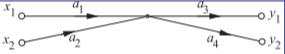\begin{aligned}y_{1}&=a_{3}(a_{1}x_{1}+a_{2}x_{2}) \\ y_{2}&=a_{4}(a_{1}x_{1}+a_{2}x_{2})\end{aligned}\nonumber

Table $$\PageIndex{1}$$: Mathematical relations in the form of signal flow graphs with edges connecting nodes. Edges and nodes are used in graph theory, a superset of SFG theory. An edge is also called a branch.Figure $$\PageIndex{2}$$: Two-port represented as (a) a two-port network with incident and reflected waves; and (b) its SFG representation.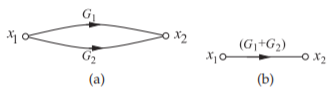Figure $$\PageIndex{3}$$: Signal flow graph representation of addition.Figure $$\PageIndex{4}$$: Cascade reduction of SFG: (a) three cascaded blocks; and (b) reduced form.Figure $$\PageIndex{5}$$: Signal flow graph simplification eliminating a variable.

reduced and simplified to arrive at a simple relationship between system input and output. Humans are extraordinarily good at pattern recognition.

Figure $$\PageIndex{3}$$ depicts SFG addition. Figures $$\PageIndex{3}$$(a and b) denote

$\label{eq:5}x_{2}=G_{1}x_{1}+G_{2}x_{1}$

### Multiplication

Consider the three cascaded blocks represented by the SFG of Figure $$\PageIndex{4}$$(a). Here the output of the first block, $$x_{2}$$, is described by $$x_{2} = G_{1}x_{1}$$. Now $$x_{2}$$ is the input to the second block with output $$x_{3} = G_{2}x_{2}$$, and so on. The total response is the product of the individual responses (see Figure $$\PageIndex{4}$$(b)):

$\label{eq:6}x_{4}=G_{1}G_{2}G_{3}x_{1}$

### Commutation

The rules that govern the simplification of SFGs use the fact that each graph represents a set of simultaneous equations. Consider the removal of the internal node, $$b_{3}$$, in Figure $$\PageIndex{5}$$(a). Here

$\label{eq:7}b_{3}=S_{31}a_{1}+S_{32}a_{2}\quad\text{and}\quad b_{4}=S_{43}b_{3}$

Node $$b_{3}$$ is called a mixed node (being both input and output) and can be eliminated, so

$\label{eq:8}b_{4}=S_{31}S_{43}a_{1}+S_{32}S_{43}a_{2}$

which has the SFG of Figure $$\PageIndex{5}$$(b). In Figure $$\PageIndex{5}$$(b) the node representing the variable $$b_{3}$$ has been eliminated. Thus elimination of a node corresponds toFigure $$\PageIndex{6}$$: Signal flow graphs having a self-loop: (a) original SFG; and (b) after eliminating $$a_{2}$$.Figure $$\PageIndex{7}$$: Signal flow graph of (a) a self-loop; and (b) with the loop eliminated.Figure $$\PageIndex{8}$$: Signal flow graph with loop.

the elimination of a variable in the simultaneous. It is sufficient to recognize the SFG pattern shown in Figure $$\PageIndex{5}$$(a) and replace it with the SFG of Figure $$\PageIndex{5}$$(b) to achieve SFG reduction.

### Self-loop

Recognizing a self-loop and eliminating it is the best example yet of pattern identification and direct application of SFG reduction strategies. Consider the SFG of Figure $$\PageIndex{6}$$(a), which has a self-loop, which describes The equations for this graph are

$\label{eq:9}b_{3} = S_{32}a_{2},\quad S_{23}a_{2} = b_{2},\quad b_{2} = S_{21}a_{1} + S_{23}a_{3},\quad a_{3} = b_{3}$

Thus

$\label{eq:10}b_{3} = S_{32}S_{21}a_{1} + S_{32}S_{23}b_{3}\quad\text{ and }\quad (1 − S_{32}) b_{3} = S_{32}S_{21}a_{1}$

where the variable $$b_{2}$$ has been eliminated. The graph of Equation $$\eqref{eq:10}$$ is shown in Figure $$\PageIndex{7}$$(a). The loop attached to node $$b_{3}$$ is called a self-loop. Such loops are not particularly convenient and can be removed by writing Equation $$\eqref{eq:10}$$ in the form

$\label{eq:11}b_{3}=\frac{S_{21}S_{32}}{1-S_{23}S_{32}}a_{1}$

and the SFG for this equation is shown in Figure $$\PageIndex{7}$$(b). The rule for removing self-loops follows from the manner in which Figure $$\PageIndex{7}$$(a) was transformed into Figure $$\PageIndex{7}$$(b). As an example, Figure $$\PageIndex{8}$$(a) becomes Figure $$\PageIndex{8}$$(b) and this can be reduced to the SFG of Figure $$\PageIndex{8}$$(c).

As a further example, consider the more difficult multiple-loop case shown in Figure $$\PageIndex{9}$$(a). This may be redrawn as Figure $$\PageIndex{9}$$(b). The two selfloops are added and then the graph reduces to that in Figure $$\PageIndex{9}$$(c).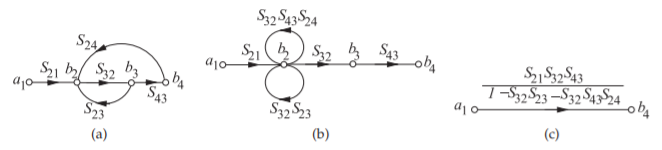Figure $$\PageIndex{9}$$: Signal flow graph with multiple loops.Figure $$\PageIndex{10}$$: Sequence of graphical manipulations in Example $$\PageIndex{1}$$ reducing a terminated two-port to a reflection only.

Example $$\PageIndex{1}$$: Signal Flow Graph

Draw the SFG of a two-port with a load (at Port $$\mathsf{2}$$) having a voltage reflection coefficient of $$\Gamma_{L}$$, and at Port $$\mathsf{1}$$ a source has a reflection coefficient of $$\Gamma_{S}$$. Using SFG analysis, derive an expression for the input reflection coefficient looking into the two-port at Port $$\mathsf{1}$$.

Solution

The two-port is shown to the right with the load attached. The input reflection coefficient is $$\Gamma_{\text{in}} = b_{1}/a_{1}$$ and the properties of the source here have no impact.Figure $$\PageIndex{11}$$

The sequence of SFG manipulations is shown in Figure $$\PageIndex{10}$$ beginning with the SFG in the top left-hand corner. So the input reflection coefficient is

$\label{eq:12}\Gamma_{\text{in}}=\frac{b_{1}}{a_{1}}=S_{11}+\frac{S_{21}S_{12}\Gamma_{L}}{1-S_{22}\Gamma_{L}}$Figure $$\PageIndex{12}$$: Development of the signal flow graph model of a source. The model in (a) is for a real reference impedance $$Z_{0}$$.

## 3.2.4 SFG Model of a Source

The SFG model, referenced to a possibly complex impedance $$Z_{0}$$, of a source with a Thevenin equivalent voltage $$E$$ and impedance $$Z_{S}$$ is shown in Figure $$\PageIndex{12}$$(a). In developing this model the circuit shown in Figure $$\PageIndex{12}$$(b) is used where the transmission line of infinitesimal length separates the forward and backward-traveling voltage waves, $$V_{1}^{+}$$ and $$V_{1}^{−}$$ for a system reference impedance of $$Z_{0}$$, the characteristic impedance of the transmission line. The SFG model used with the transmission line-augmented source is shown in Figure $$\PageIndex{12}$$(c). The SFG model of Figure $$\PageIndex{12}$$(c) describes

$\label{eq:13}a_{1}=b_{s}+\Gamma_{S}b_{1}$

where the source reflection coefficient $$\Gamma_{S} = (Z_{S} − Z_{0})/(Z_{S} + Z_{0})$$. The circuit equations for Figure $$\PageIndex{12}$$(b) are

$\label{eq:14}V_{1}=E-I_{S}Z_{S}=V_{1}^{+}+V_{1}^{-}\quad\text{and}\quad I_{S}=\frac{V_{1}^{+}}{Z_{0}}-\frac{V_{1}^{-}}{Z_{0}}$

Now, the traveling wave voltages are related to the a and b root power waves as (see Equation $$\eqref{eq:15}$$)

$\label{eq:15}a_{1}=\frac{V_{1}^{+}}{\sqrt{\Re\{Z_{0}\}}}\quad\text{and}\quad b_{1}=\frac{V_{1}^{-}}{\sqrt{\Re\{Z_{0}\}}}$

Then combining Equations $$\eqref{eq:14}$$ and $$\eqref{eq:15}$$ yields

\begin{align}E&=V_{1}^{+}\frac{Z_{S}+Z_{0}}{Z_{0}}-V_{1}^{-}\frac{Z_{S}-Z_{0}}{Z_{0}}\nonumber \\ \frac{Z_{0}}{\sqrt{\Re\{Z_{0}\}}}\frac{1}{Z_{S}+Z_{0}}E&=\frac{V_{1}^{+}}{\sqrt{\Re\{Z_{0}\}}}\left(\frac{Z_{S}+Z_{0}}{Z_{S}+Z_{0}}\right)-\frac{V_{1}^{-}}{\sqrt{\Re\{Z_{0}\}}}\left(\frac{Z_{S}-Z_{0}}{Z_{S}+Z_{0}}\right)\nonumber \\ \label{eq:16}\frac{Z_{0}}{\sqrt{\Re\{Z_{0}\}}}\frac{1}{Z_{S}+Z_{0}}E&=a_{1}-b_{1}\Gamma_{S}\end{align}

Comparing Equations $$\eqref{eq:13}$$ and $$\eqref{eq:16}$$ it is seen that

$\label{eq:17}b_{s}=\frac{Z_{0}}{\sqrt{\Re\{Z_{0}\}}}\frac{1}{Z_{S}+Z_{0}}E$

Thus the SFG model of a source is as shown in Figure $$\PageIndex{12}$$(a) where the model is shown for a real $$Z_{0}$$.

Example $$\PageIndex{2}$$: Power Delivered to a Load and Substitution Loss

This example illustrates the method for determining powers using signal flow graph analysis with generalized $$S$$ parameters having reference impedances $$Z_{01}$$ at Port $$\mathsf{1}$$ and $$Z_{02}$$ at Port $$\mathsf{2}$$. This is then used to determine substitution loss. The substitution loss is the ratio of the power delivered to a load with an initial two-port, to the power delivered with the substituted final two-port network. The SFG elements are shown on the right with $$\Gamma_{S}$$ referred to $$Z_{01}$$ and $$\Gamma_{L}$$ referred to $$Z_{02}$$.Figure $$\PageIndex{13}$$

Solution

The SFG manipulations that determine the power delivered to the load are shown in Figure $$\PageIndex{14}$$ where

$\label{eq:18}\alpha=\frac{S_{21}}{1-S_{22}\Gamma_{L}},\quad\beta=\frac{1}{1-\Gamma_{S}(S_{11}+S_{12}\Gamma_{L}\alpha)}$

and

\begin{align}b_{2}&=b_{S}\alpha\beta =b_{S}\frac{S_{21}}{(1-S_{22}\Gamma_{L})}\frac{1}{\{1 − \Gamma_{S} [S_{11} + S_{12}S_{21}\Gamma_{L}/(1 − S_{22}\Gamma_{L})]\}}\nonumber \\ &=b_{S}\frac{S_{21}}{(1-S_{22}\Gamma_{L})}\frac{(1-S_{22}\Gamma_{L})}{[(1 − S_{22}\Gamma_{L}) − S_{11}\Gamma_{S}(1 − S_{22}\Gamma_{L}) + S_{12}S_{21}\Gamma_{S}\Gamma_{L}]}\nonumber \\ &=b_{S}\frac{S_{21}}{1 − S_{22}\Gamma_{L} − S_{11}\Gamma_{S} + S_{11}S_{22}\Gamma_{S}\Gamma_{L} + S_{12}S_{21}\Gamma_{S}\Gamma_{L}}\nonumber \\ \label{eq:19}&=b_{S}\frac{S_{21}}{(1 − S_{11}\Gamma_{S})(1 − S_{22}\Gamma_{L}) − S_{12}S_{21}\Gamma_{S}\Gamma_{L}}\end{align}

Then the power delivered to the load is (using Equation (2.4.9))

$\label{eq:20}P_{L}=\frac{1}{2}|b_{2}|^{2}(1-|\Gamma_{L}|^{2})=\frac{\frac{1}{2}|b_{S}S_{21}|^{2}(1-|\Gamma_{L}|^{2})}{|(1-S_{11}\Gamma_{S})(1-S_{22}\Gamma_{L})-S_{12}S_{21}\Gamma_{S}\Gamma_{L}|^{2}}$

The substitution loss, $$L_{S}$$, is the ratio of the power delivered to the load by an initial two-port identified by the leading superscript ‘$$i$$’, and the power delivered to the load with a final two port identified by the leading superscript ‘$$f$$’. In decibels

$\label{eq:21}L_{S}|_{\text{dB}}=10\log\left|\frac{^{i}P_{L}}{^{f}P_{L}}\right|=10\log\left|\frac{[(1 −\:^{f}S_{11}\Gamma_{S})(1 −\:^{f}S_{22}\Gamma_{L}) −\:^{f}S_{12}\:^{f}S_{21}\Gamma_{S}\Gamma_{L}]}{[(1 −\:^{i}S_{11}\Gamma_{S})(1 −\:^{i}S_{22}\Gamma_{L}) −\:^{i}S_{12}\:^{i}S_{21}\Gamma_{S}\Gamma_{L}]}\right|^{2}$

Substitution loss is used in deriving formulas for insertion loss, see Section 2.8.2.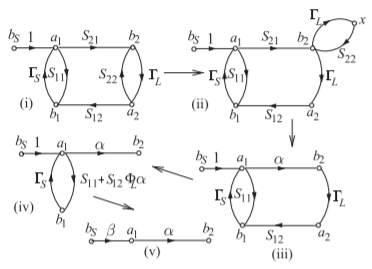Figure $$\PageIndex{14}$$: SFG manipulations in developing the power delivered to a load with a two-port network between source and load.

## 3.2.5 Mason's Rule

Mason’s rule is a general procedure for reducing SFGs with multiple loops and is a systematic procedure for the reduction of SFGs to a single branch. Mason  derived the formula with the aim of developing a general method for the computer analysis of electrical circuits. First, a number of topology definitions must be introduced.

A path begins at one node and traverses a number of successive edges in the direction of the arrows to arrive at the final node or sink. In SFG analysis, a path is a forward path, as the successive edges are traversed in the direction of the arrows. An open path encounters the same node only once, and a closed path (or a loop) ends on the same node at which it is started. The product of the transmittances of all edges in a loop is called the loop transmittance. Two paths, open or closed, are said to be non-touching paths if they have no nodes in common. Similarly, disjoint loops are loops that have no nodes in common.

Mason's rule:

If $$T$$ represents the overall graph transfer function and $$T_{k}$$ represents the transfer function of the $$k$$th forward path from source to sink, then

$\label{eq:22}T=\frac{1}{\lambda}\sum_{k}T_{k}\lambda_{k}$

where $$\lambda$$ is the determinant of the matrix of coefficients of the equations represented by the SFG (usually called the determinant of the graph) and $$\lambda_{k}$$ is the determinant of that part of the graph (subgraph) that does not touch the $$k$$th forward path. The determinant is given by

$\label{eq:23}\lambda=1-\sum_{k}P_{j1}+\sum_{j}P_{j2}-\sum_{j}P_{j3}+\ldots$

where the first summation in Equation $$\eqref{eq:23}$$ is the sum of the loop transfer functions of all the loops in the graph. In the second summation, the products of the transfer functions of all pairs of non-touching loops are added. In the third summation, the products of the transfer functions of disjoint loops taken three at a time are added, and so on. Mason’s rule can be quite difficult to apply, but any problem that Mason’s rule can solve can also be solved using multiple applications of SFG manipulations described previously.

Example $$\PageIndex{3}$$: Application of Mason's Rule

Use Mason’s rule to reduce the SFG shown in Figure $$\PageIndex{15}$$(a). Here, input node $$a_{1}$$ is the excitation and the output node $$b_{4}$$ is the response. The transmittance $$T$$ is equal to $$b_{4}/a_{1}$$.

Solution

First, consider the determinant of the flow graph. Since all the loops have at least one node in common, the expression for the determinant reduces to

$\label{eq:24}\lambda=1-\sum_{j}P_{j1}$

There are three loops identified in Figure $$\PageIndex{15}$$(b) with transmittances $$\mathsf{A} = S_{21}S_{32}S_{13},\: \mathsf{B} = S_{32}S_{43}S_{24},$$ and $$\mathsf{C} = S_{21}S_{32}S_{43}S_{14}$$. Thus the sum of the loop transmittances is

$\label{eq:25}\sum_{j}=P_{j1}=S_{21}S_{32}S_{13} + S_{32}S_{43}S_{24} + S_{21}S_{32}S_{43}S_{14}$

and the determinant is given by

$\label{eq:26}\lambda= 1 − S_{21}S_{32}S_{13} − S_{32}S_{43}S_{24} − S_{21}S_{32}S_{43}S_{14}$

This rule works well with the human ability to recognize patterns, in this case loops. There is only one forward path, identified in Figure $$\PageIndex{15}$$(b), from source $$a_{1}$$ to sink $$b_{4}$$, given by

$\label{eq:27}T_{1}=S_{21}S_{32}S_{43}$

Since all the loops of the graph have nodes that touch $$T_{1}$$, then $$\lambda_{1} = 1$$ and

$\label{eq:28}\sum_{k}T_{k}\lambda_{k}=T_{1}\lambda_{1}=S_{21}S_{32}S_{43}$

Thus

$\label{eq:29}\frac{b_{4}}{a_{1}}=T=\frac{S_{21}S_{32}S_{43}}{1− S_{21}S_{32}S_{13} − S_{32}S_{43}S_{24} − S_{21}S_{32}S_{43}S_{14}}$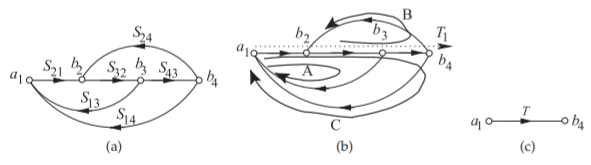Figure $$\PageIndex{15}$$: Signal flow graph used in Example 3.2.3 applying Mason’s rule: (a) SFG; (b) annotation identifying loops and through-path; and (c) final reduction.

## 3.2.6 Summary

SFG reduction can be used with numerical values but the real power comes from the ability to develop symbolic expressions. In RF and Microwave engineering these expressions nearly always involve scattering parameters but they can be used with any sets of equations. Design is best undertaken by developing symbolic solutions as these enable optimization to be performed and insights gained as to the significance of parameters.

This page titled 3.2: Signal Flow Graph is shared under a CC BY-NC license and was authored, remixed, and/or curated by Michael Steer.